# MRI Brain Tumor Identification and Classification Using Deep Learning Techniques

MRI Brain Tumor Identification and Classification Using Deep Learning Techniques
Department of Computer Science, Ferhat Abbas University of Setif, Setif 19000, Algeria

LMSE Laboratory: University Mohamed El Bachir El Ibrahimi of Bordj BouArreridj, Bordj Bou Arreridj 34000, Algeria

Department of Computer Science, University Mohamed El Bachir El Ibrahimi of Bordj BouArreridj, Bordj Bou Arreridj 34000, Algeria

Department of Network and Computer Security, State University of New York Polytechnic Institute, Utica, NY 13502, USA

Corresponding Author Email:
hafidachellakh@gmail.com
Page:
13-22
|
DOI:
https://doi.org/10.18280/isi.280102
22 December 2022
|
Accepted:
30 January 2023
|
Published:
28 February 2023
| Citation

OPEN ACCESS

Abstract:

Deep learning has exponentially enhanced the state-of-the-art in several Artificial Intelligence (AI) domains, including computer vision, user authentication, healthcare, object recognition and image processing. Recently, deep rule-based classifier (DRB) is being employed to solve diverse problems of classification or prediction. Thus, in this paper, we present a novel, simple, automatic, and effective DRB classifier-based scheme for MRI brain tumor classification. The proposed framework is composed of three stages, i.e., preprocessing, feature extraction and classification. Especially, in the second stage, we have investigated and analyzed comparative performances of various deep features extracted by the AlexNet, VGG-16, ResNet-50, ResNet-18 deep learning networks. After feature extraction step, a DRB classifier is employed for classification. The proposed method is evaluated on two publicly available datasets that are available on Kaggel website. The first database is a binary database (i.e., tumor and no tumor). Whereas the second one is a multiclass database (i.e., Meningioma, Glioma and Pituitary tumor). Experimental results show that the proposed method can obtain notable performances. Moreover, the comparative study with classical methods (e.g., SVM, KNN, Decision tree) as well as several state-of-the-art distance techniques demonstrated the effectiveness of proposed approach in MRI brain tumor detection and classification.

Keywords:

brain tumor detection, classification, deep learning, feature extraction

1. Introduction

According to the World Health Organization (WHO), cancer is one of the leading grounds of death worldwide [1, 2]. Unlike cancer, a tumor could be benign or malign . The uniformity structures and non-active cancer cells are present in benign tumor. But non-uniformity structures and active cancer cells, which have been spread or could spread all various parts, are present in malignant tumor. The key issue is early detection and classification of brain tumor, thereby most suitable therapy, radiation, surgery, or chemotherapy can be decided to avoid the further complications . Consequently, the chances of survival of a tumor-infected patient can be increased significantly .

Owing to the high soft tissue’s contrast, high spatial resolution, and non-invasive characteristic, MRI is the most effective technique for diagnosing human brain tumors . However, it is still a challenging and time-consuming process because of the brain tumors’ complexity. The manual evaluation of results and images depends strongly on the radiologists’ experience and knowledge. Furthermore, conventional methods are impractical for large amounts of data, and are also non-reproducible and susceptible to human subjectivity. Therefore, computer-aided-diagnosis (CAD) systems are very necessary to overcome such limitations.

Over the years, automated machine learning based methods have been developed. However, most traditional machine learning approaches have certain limitations with MRI images, mainly owing to huge data, and so on. New developments in AI show that deep learning (DL) frameworks can easily handle and processes big data efficiently. In particular, recent deep learning techniques have achieved great success and popularity not only in healthcare/medical fields but also in several AI domains such as autopilot vehicles, speech recognition, image classification, and hand-written digit recognition. The success of DL is due to the large amount of available data and compute capacity. Such factors allow DL frameworks to effectively learn and extract powerful features from data; rather than classical approaches that use hand-crafted features or rules designed by experts. Also, numbers of DL studies have demonstrated results surpassing human accuracy.

The latest introduction of information technology in medical diagnosis is greatly helping specialists to offer better solutions. These days, early-stage brain tumors detection is possible and vital for efficient treatments. The problem of brain tumor classification can mainly be divided into two categories: (i)binary classification, i.e., normal and abnormal classes; (ii) multi classification, which aims at discriminating between different types of brain tumors such as Glioma, Meningioma, Pituitary, and Metastatic . Despite advances in MRI brain tumor detection and classification using machine learning methods, the performances sometime are less than expected if the size of the database is small or the framework is huge and requires huge database and compute facilities.

Inspired by such issues and to solve some of the existing problems, we propose a new automated MRI brain tumor classification framework based on deep learning techniques. It consists of three stages, i.e., preprocessing, feature extraction and classification. Both feature extraction and classification steps are hinged on deep learning techniques. Namely, the proposed work extracts deep features from the MRI images, and utilizes the deep rule based (DRD) classifier for the classification of MRI brain tumor. The proposed method is evaluated on two public datasets. The first database is a binary database (i.e., tumor, no tumor) and the second one is a multiclass database (i.e., Meningioma, Glioma and Pituitary tumor).

The main contributions of this study are summarized as follows:

• A generalized framework for MRI brain tumor classification using deep learning techniques.
• For enhanced classification performance, use of deep features with the DRB classifier.
• Several experiments on two public datasets, i.e., a binary database (i.e., tumor, no tumor), and a multiclass database (i.e., Meningioma, Glioma and Pituitary tumor). Furthermore, a comparative analysis of results on a small database and a large database.
• A comparative evaluation of the state-of-the-art classical methods (SVM, KNN, Decision tree) together with different low- and high-level features.
• A comparative study of various distance methods with the DRB classifier.

The remained of this paper is organized as follows. Section 2 presents an overview of related work. The proposed methodology is presented in Section 3. Section 4 describes the architecture of the DRB classifier employed in this paper. Experimental results are presented in Section 5. Conclusions are drawn in Section 6.

2. Related Work

There exist several studies on detection and classification of MRI brain images using machine learning techniques, summarized in Table 1; such as fuzzy clustering means (FCM), K-nearest neighborhood (K-NN), and support vector machine (SVM). For instance, Zhang et al.  proposed pathological brain detection (PBD) method, where wavelet packet Tsallis entropy (WPTE) technique and fuzzy support vector machine (FSVM) has been applied in feature extraction and classification step, respectively. Bahadure et al.  combined Berkeley Wavelet Transformation (BWT) features with the support vector machine (SVM) to classify healthy and infected tissues from MRI images. They achieved an accuracy of 96.51%. Zhang et al.  proposed an automatic method for classification of MRI brain images based kernel support vector machine (KSVM) and wavelet transform (WT) features with Principal Component Analysis (PCA) to reduce the size of features. Usman and Rajpoot  investigated wavelet texture features with random forest classifier to predict tumor labels as multiclass classification. Cheng et al.  presented an automatic classification method by focusing on the classification of three types of brain tumors (i.e., Meningioma, Glioma, and Pituitary tumor). To improve the classification accuracy, the augmented tumor region was used instead of the original image. For feature extraction, three methods (i.e., intensity histogram, gray level, and co-occurrence matrix (GLCM), and bag-of-words) were combined.

In recent years, several studies using deep learning (DL) techniques have been developed. For example, Ari and Hanbay  designed a technique based on extreme learning machine local receptive fields (ELM-LRF) to classify the tumor region as benign or malignant, which obtained an accuracy of 97.18%. Varuna et al.  presented Discrete Wavelet Transformation (DWT) for MRI brain images feature extraction and probabilistic neural network as classifier, and achieved nearly 100% accuracy. Byale et al.  presented a binary classification technique. The proposed technique first employed Grey Level Co-occurrence Matrix (GLCM) for feature extraction followed by Neural Networks (NN) to classify the tumor as benign or malignant with accuracy of 93.33%. Badža et al.  presented a new CNN architecture for MRI-based brain tumor classification. They examined the classification of three tumor types (i.e., Meningioma, Glioma and Pituitary tumor) from an imbalanced database. The accuracy achieved by this work was 96.56%.

Table 1. Summary of prior works on MRI brain tumor identification and classification system

 Year Authors Features Methods Accuracy 2020 Badža et al.  CNN CNN 96.56% 2018 Varuna et al.  Discrete wavelet Transformation (DWT) Probabilistic Neural Network 100% 2015 Cheng et al.  Intensity histogram, Gray Level Co-occurrence Matrix (GLCM), and Bag-of-Words (BoW) model SVM 91.14% 2015 Zhang et al.  Wavelet Packet Tsallis Entropy (WPTE), Fuzzy Support Vector Machine (FSVM) 99.49% 2017 Bahadure et al.  Berkeley Wavelet Transformation (BWT) Support Vector Machine (SVM) 96.51% 2012 Zhang and Wu  Wavelet transform (WT) followed by Principal Component Analysis (PCA) Kernel Support Vector Machine (KSVM) 99.38% 2017 Usman and Rajpoot  Wavelet Texture Features Random forest classifier 95.00% 2018 Ari and Hanbay  Convolutional Neural Network (CNN) Extreme Learning Machine Local Receptive Fields (ELM-LRF) 97.18% 2018 Byale et al.  Grey Level Co-occurrence Matrix GLCM Neural Networks (NN) 93.33%
3. Proposed Methodology

The main aim of this study is to devise an MRI brain tumor classification method using deep learning techniques. The proposed methodology which presented in Figure 1 it consists of three stages, i.e., preprocessing, feature extraction, and classification. The feature extraction and classification steps are both based on deep learning techniques.

## 1.png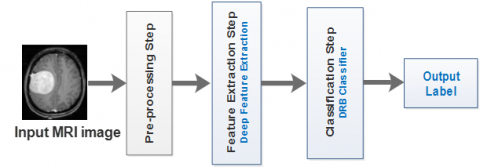Figure 1. Block diagram of the presented method

3.1 Pre-processing step

The preprocessing step plays an important role in improving the quality of the image that leads to achieving better results in feature extraction and classification steps. It consists of fundamental pre-processing techniques such as binarization, normalization, rotation, resizing, and removal of undesired parts of MR images.

3.2 Feature extraction step

Feature extraction is also a vital step in the classification process. It consists of finding the most significant characteristics from the original data in order to improve the overall efficiency of the system. To this aim, in this study, we employ deep learning feature descriptors. We utilized four pre-trained convolution neural networks such as AlexNet, VGG-16, ResNet-18, and ResNet-50. These pre-trained CNN networks have been utilized to extract suitable MRI image features.

3.2.1 AlexNet

AlexNet, introduced by Krizhevsky et al. , and competed in the ImageNet challenge in 2012. The network achieved a top-5 error of 16.4%. AlexNet includes five convolutional layers, three max pool layers, and three fully connected layers. The input image size should be [227 × 227 × 3] [15, 16].

3.2.2 VGG-16

VGG (Visual Geometry Group Net) is convolutional neural network (CNN) introduced by Simonyan and Zisserman . It was one of the most prominent models that participated in ILSVRC-2014 (ImageNet Large Scale Visual Recognition Competition) for the classification task. VGG Net was trained on ImageNet database which contains over 14 million images of 1000 classes (i.e., 1.3 million images for training, 50,000 images for validation, and 100,000 images for testing). The model achieved an accuracy of 92.7% on ImageNet database. VGG Net input image size should be 224×224 RGB. The images are then passed through a stack of convolutional layers with the fixed filter size of 3×3 and the stride of 1. VGG-16 architecture contains five max pooling layers integrated through a stack of convolutional layers that are followed by 3 fully connected layers. The first and second layers have 4096 channels, while the third one has 1,000 channels. Soft-max layer is the final layer in VGG-16 model .

3.2.3 Residual Network (ResNet-50 and ResNet-18)

ResNet is an artificial neural network (ANN) developed by He et al. in 2016. It ranked 1st in ILSVRC 2015 with top 5 error rate of 3.57%. Also, it won the ILSVRC and COCO 2015 competitions for ImageNet Discovery, ImageNet Localization, Coco Discovery, and Coco Segmentation. It is a very deep feed forward neural network with hundreds of layers, much deeper than previous neural networks. Skip connections or shortcuts are used to jump over some layers. ResNet gives a relative improvement of 28% over VGG-16. The ResNet18 makes a good offset between depth and performance. It contains five convolutional layers, one average pooling, a fully-connected layer, and finally a softmax layer. ResNet50 is composed by 49 convolutional layers and a fully-connected layer at the end of the network .

3.3 Classification step

There exist several techniques for classification of data such as fuzzy clustering means (FCM), support vector machine (SVM), and artificial neural network (ANN).

Motivated by the high classification accuracy achieved by the DRB classifier , we explore it for the classification of MRI brain tumors.

4. General Architecture of the DRB Classifier

As shown in Figure 2, the proposed method is composed of three main stages, i.e., pre-processing, feature extraction, and classification using DRB block (i.e., massively parallel rules, decision-maker). The pre-processing block and feature extraction layer are described in Sections 3.1 and 3.2, respectively. In the following, we describe the DRB classification.

## 2.png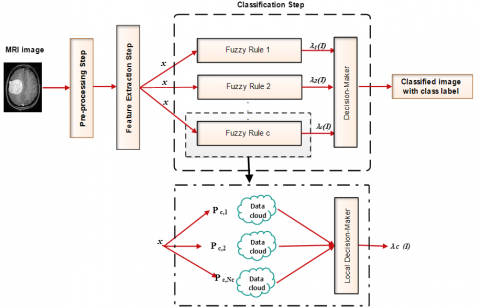Figure 2. General architecture of the DRB classifier

DRB block consists of two sections. The first section is basis of the DRB classifier for training phase. It is a series of parallel IF...THEN rules which are based on the self-developed FRB models known as AnYa type. These non-parametric rules do not need the existence of a membership function to be defined [21, 22]. They emerge from data patterns by following the concept of the Empirical Data Analytics . The form of each fuzzy rule, as presented in Table 3, is a disjunction (logical operators OR) between a considerable number of fuzzy sets predetermined by many prototypes which are the most representative of the data clouds.

The second section is the decision-maker which is used during the validation stage. It is the process that, based on the operator “winner-takes-all”, determines the label of winning class. The one making the last call on the basis of the results is the local decision-maker [24, 25]. For more precision, we present the key notations with their respective definitions used in this paper in Table 2.

Table 2. Descriptions of the key notations used in this paper

 Notations Description C The number of Dataset classes d Feature vector dimensionality k Observed training images number I A single input image x The corresponding feature vector of I $\bar{x}$ Vector normalization Nc The number of identified prototypes of the Cth class μc The global mean of feature vectors of the training images of the Cth class D Data density Ic,k The kth training image of the Cth class xc,k The corresponding feature vector of Ic,k Pc,i The ith prototype of the Cth class pc,i The mean of feature vectors of the training images associated with Pc,i Sc,i The number of training images associated with Pc,i rc,i The radius of the area of influence of the dataclouds d associated with Pc,i λc The score of confidence given by the local decision-maker of the Cth fuzzy rule Sgi The ith segment of the image I or local information

Table 3. Samples of AnYa type fuzzy rules identified with brain tumor dataset

## biao_3.png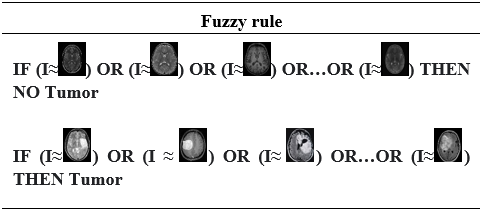4.1 Massively parallel FRB

The fuzzy rule-based (FRB) layer is a group of IF…THEN… rules, which are extremely parallel based on the so-called AnYa type 0-order fuzzy rules . These non-parametric rules do not need the existence of a membership function to be defined [21, 22]. After the Empirical Data Analytics concept, the fuzzy rules emerge from data patterns .

$\mathrm{IF}\left(\mathrm{I} \sim \mathrm{P}_{\mathrm{c}, 1}\right) \mathrm{OR} \ldots \mathrm{OR}\left(\mathrm{I} \sim \mathrm{P}_{\mathrm{c}, \mathrm{N}_{\mathrm{c}}}\right)$ THEN (class $\mathrm{C} )$     (1)

where, “~” means resemblance that can be considered as a fuzzy degree of satisfaction/membership  or typicality [21, 25]; I is a specific image; c=1, 2, ..., C; Nc is the number of prototypes of the Cth class. The identified prototypes are denoted by P.

4.1.1 Training process of the DRB system

In this section, a summary of the main procedure of the training process of a particular FRB subsystem is outlined. Because of the highly parallel structure of the DRB system, we consider the Cth (c=1, 2, …, C) fuzzy rule.

We initialize the kth (k←1) of the training image to check the condition 1 and separate stage 0 and the other stages.

Condition 1:

$I F(k=1)$ THEN (startwith stage 0)     (2)

If condition 1 is satisfied, then it is the first image arrived then we initialize the system by following the stage 0.

If condition 1 is not met, then the system has been initialized before and we pass directly the stage 1.

Stage 0: System Initialization.

We initialize the Cth fuzzy rule by the first image Cth (denoted by Ic,1) of the corresponding class with the global feature vector denoted by xc,1(xc,1=[xc,1,1, xc,1,2, xc,1,d]), d is the dimensionality. Then, we initialize the meta-parameters of the system as follows:

$\begin{gathered}k \leftarrow 1 ; \boldsymbol{\mu}_c \leftarrow \overline{\boldsymbol{x}}_{c, 1} ; N_c \leftarrow 1 ; \mathbf{P}_{c, N_c} \leftarrow \mathbf{I}_{c, 1} ; \boldsymbol{p}_{c, N_c} \\ \leftarrow \overline{\boldsymbol{x}}_{c, 1} ; S_{c, N_c} \leftarrow 1 ; r_{c, N_c} \leftarrow r_c ;\end{gathered}$     (3)

where, k represents the current time instance, μc is the global mean of all the observed data samples of the Cth class. $\boldsymbol{p}_{c, N_c}$ is the mean of feature vectors of the images associated with the first datacloudwith the visual prototype $\mathbf{P}_{c, N_c}, S_{c, N_c}$ is the number of images associated with the data cloud. $r_{c, N_c}$ is the radius of the area of the data cloud, $r_0$ is a small value to stabilize the initial status of the newly formed data clouds.

Stage 1: System preparation.

In this stage, we calculate the densities to check the condition 2. Firstly, we read the newly arrived kth(kk+1) of the training image (Ic,k) that belongs to the Cth class. Then, we update the global mean μc and calculate the data densities of all existing prototypes Pc,i by following:

$\boldsymbol{\mu}_c \leftarrow \frac{k-1}{k} \boldsymbol{\mu}_c+\frac{1}{k} \overline{\boldsymbol{x}}_{c, k}$     (4)

$D\left(\mathbf{P}_{c, i}\right)=\frac{1}{1+\left\|\boldsymbol{p}_{c, i}-\boldsymbol{\mu}_c\right\|^2 / \sigma_c^2}$      (5)

$D\left(\mathbf{I}_{c, k}\right)=\frac{1}{1+\left\|\overline{\boldsymbol{x}}_{c, k}-\boldsymbol{\mu}_c\right\|^2 / \sigma_c^2}$      (6)

where, $\sigma_c^2=1-\left\|\boldsymbol{\mu}_c\right\|^2$.

Stage 2: System update.

In this stage, we check the condition 2, if the Ic,k becomes a new prototype or we find the nearest prototype to Ic,k using the D(Pc,i) and D(Ic,k) calculated in the previous stage. Then, we update the system and meta-parameters.

Condition 2:

$\begin{gathered}\operatorname{IF}\left(D\left(\mathbf{I}_{c, k}\right)>\max _{i=1,2,3, \ldots, N_c}\left(D\left(\mathbf{P}_{c, i}\right)\right)\right) \operatorname{OR}\left(D\left(\mathbf{I}_{c, k}\right)<\min _{i=1,2, \ldots, N_c}\left(D\left(\mathbf{P}_{c, i}\right)\right)\right) \text { THEN } \left(I_{c, k} \text { is new prototype }\right)\end{gathered}$     (7)

If condition 2 is met, then Ic,k is new prototype with new data cloud.

$\begin{gathered}N_c \leftarrow N_c+1 ; \mathbf{P}_{c, N_c} \leftarrow \mathbf{I}_{c, 1} ; P_{c, N_c} \leftarrow \overline{\boldsymbol{x}}_{c, 1} ; \\ S_{c, N_c} \leftarrow 1 ; r_{c, N_c} \leftarrow r_c ;\end{gathered}$      (8)

If condition 2 is not satisfied, we find the nearest prototype Pc,n to Ic,k following the Eq. (9):

$\mathbf{P}_{c, n}=\arg \min _{j=1,2, \ldots, N_c}\left(\left\|\overline{\boldsymbol{x}}_{c, k}-\boldsymbol{P}_{c, j}\right\|\right)$     (9)

Before we associate the Ic,k with the data cloud of Pc,n, we need to check the last condition 3 to see whether Ic,k is located in the area of influence of Pc,n:

Condition 3:

$\operatorname{IF}\left(\left\|\overline{\boldsymbol{x}}_{c, k}-\boldsymbol{p}_{c, n}\right\| \leq r_{c, N_c}\right) \operatorname{THEN}\left(\mathbf{I}_{c, k}\right.$ is assigned to $\left.\mathbf{P}_{c, n}\right)$     (10)

If the condition 3 is met, then we update the meta-parameters and Ic,k assigned to the data cloud of the prototype $\mathbf{P}_{c, \mathrm{n}}$ using:

$S_{c, n} \leftarrow S_{c, n}+1 ; \boldsymbol{p}_{c, n} \leftarrow \frac{S_{c, n}-1}{S_{c, n}} \boldsymbol{p}_{c, n}+\frac{1}{S_{c, n}} \overline{\boldsymbol{x}}_{c, k} ;$       (11)

If the condition 3 not met, then Ic,k is out of the influence area of the nearest data cloud, we consider Ic,k is a new prototype by following Eq. (8).

Once Stage 2 has been finished, the DRB system will update the fuzzy rule accordingly following the Eq. (12). Then, the K will increment by 1 (kk+1), then the system goes back to Stage 1 and read the next image and start a new processing cycle.

Stage 3: Generate Fuzzy rule based (FRB).

After all the training data has been processed, the system will generate one fuzzy rule (Rulec) based on the identified prototypes. Samples of AnYa type fuzzy rules identified with brain tumor dataset are presented in Table 3.

$Rule _c$$:\operatorname{IF}\left(\mathbf{I} \sim \mathbf{P}_{c, 1}\right) O R \ldots O R\left(\mathbf{I} \sim \mathbf{P}_{c, N_c}\right) \operatorname{THEN}(\operatorname{Class} C)$      (12)

4.1.2 Validation process of the DRB system

At end of the training process, the DRB system generates C fuzzy rules depending to the C classes. For each testing image, the system generates c score of confidence λc(I) by its local (per rule) decision-maker based on the feature vector of I, denoted by x:

$\lambda_c(\mathbf{I})=\arg \max _{j=1,2, \ldots, N_c}\left(\exp \left(-\left\|x-\boldsymbol{p}_{c, j}\right\|^2\right)\right)$      (13)

Therefore, we have C scores of confidences λc(I) = [λ1(I), λ2(I), λ3(I), …, λc(I)] for each image. These scores represent the inputs of the overall decision-maker of the DRB classifier (the last layer in Figure 2), which decides the label of the testing image using the “winner-takes-all” principle as follows:

$\operatorname{label}(\mathbf{I})=\arg \max _{c=1,2, \ldots, c}\left(\lambda_c(\mathbf{I})\right)$       (14)

The pseudo code of the training process is as follows.

 Algorithm 1. Training process of the deep rule-based classifier K=1; While the new feature vector xc,k of the kth image Ic,k of the Cth class is available Do IF (K=1) THEN Initialization using Eq. (3); Generate the Anya type fuzzy rule Eq. (12); ELSE Update μc using Eq. (4); Calculate D(Pc,i) and D(Ic,k) using Eqns. (5, 6); If (condition 2 is met) then Initialize a new data cloud using Eq. (8); Else Find Pc,n using Eq. (9); If (condition 3 is met) then Update the existing data cloud using Eq. (11); Else Initialize a new data cloud using Eq. (8); End if End if       Update the Anya type fuzzy rule using Eq. (12); End if K=k+1; End while
5. Experiments and Results

5.1 Database

In this work, we used two public datasets available on the Kaggle website. As described in Table 4, the first dataset contains 253 images with 2 classes (i.e., no tumor, pathological), the second dataset contains 3264images with 4 classes (i.e., Glioma tumor, Meningioma tumor, Pituitary tumor, No tumor). Data for no tumors were obtained from the Novoneel Chakraborty Kaggle data set. (i.e., https://www.kaggle.com/datasets/navoneel/brain-mri-images-for-brain-tumor-detection).

Table 4. Datasets descriptions

 Dataset Classes Number of images Images type Dataset 1 Tumor 155 JPG No tumor 98 Dataset 2 Glioma tumor 926 JPG Meningioma tumor 937 No tumor 500 Pituitary tumor 901

5.2 Performance metrics

To demonstrate and validate the performance of the proposed scheme, three metrics have been used, including accuracy, specificity and sensitivity calculated by Eqns. (15, 16 and 17) .

Accuracy: $\frac{\mathrm{TP}+\mathrm{TN}}{\mathrm{TP}+\mathrm{TN}+\mathrm{FP}+\mathrm{FN}}$       (15)

Sensitivity: $\frac{\mathrm{TP}}{\mathrm{TP}+\mathrm{FN}}$      (16)

Specificity: $\frac{\mathrm{TP}}{\mathrm{TP}+\mathrm{FN}}$        (17)

5.3 Experiment results

In this section, we present an experimental evaluation of the proposed deep features-based MRI brain tumor classification system. Several experiments were conducted as shown in Figure 3 by applying the pre-trained CNN models (i.e., AlexNet, VGG-16, ResNet-50, and ResNet-18) for feature extraction. The obtained results were compared with SVM, KNN and Decision Tree. All in all, the performances were assessed in terms of accuracy, sensitivity, specificity, precision, and F-measure, which are presented in Table 5, Table 6, Table 7 and Table 8. As shown in Table 5 to 8, the proposed approach gives better results with the second database (i.e., multi-class classification). An accuracy of 79.19% was obtained with AlexNet, 81.73% with VGG-16, 78.17% with ResNet50, and 80.46% with ResNet18 that surpassed SVM, KNN and decision tree techniques. For the first database (i.e., binary classification), the proposed approach gives best results with an accuracy of 82.95% with ResNet50 and 85.22 % with ResNet18. Also, one can see the proposed method’s efficacy in Figures 4-11.

## 3.png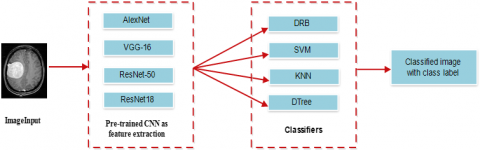Figure 3. Experimental evaluation of the proposed system

Experiment 1: AlexNet with 4 different classifiers.

## 4-1.pngDataset1

## 4-2.png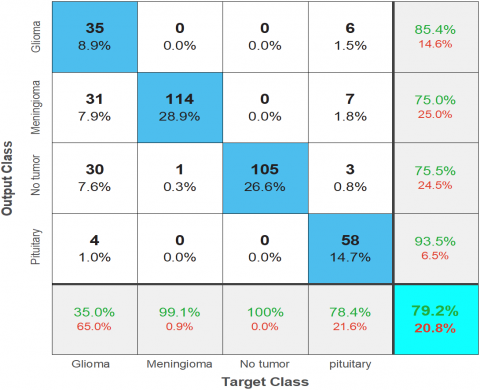Dataset 2

Figure 4. Confusion matrix of DRB with data 1 and Data 2

Experiment 2: VGG-16 with 4 different classifiers.

## 5-1.png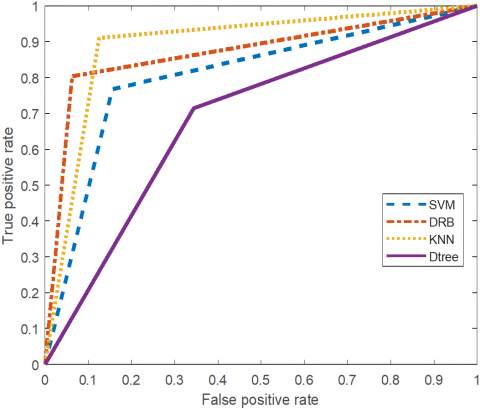Dataset 1

## 5-2.png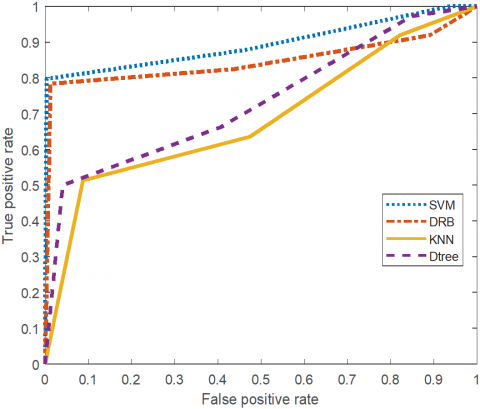Dataset 2

Figure 5. ROC curves of dataset 1 and dataset 2

## 6-1.png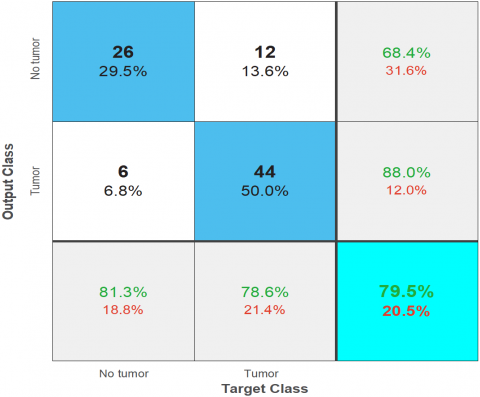Dataset 1

## 6-2.png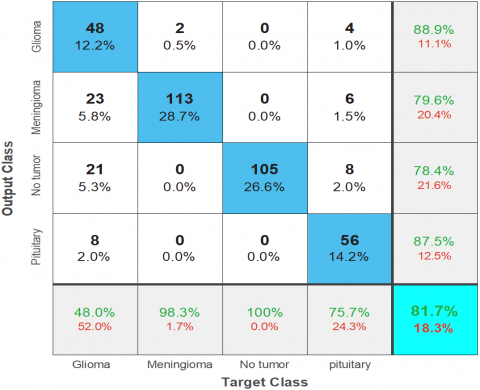Dataset 2

Figure 6. Confusion matrix of DRB with data 1 and Data 2

## 7-1.png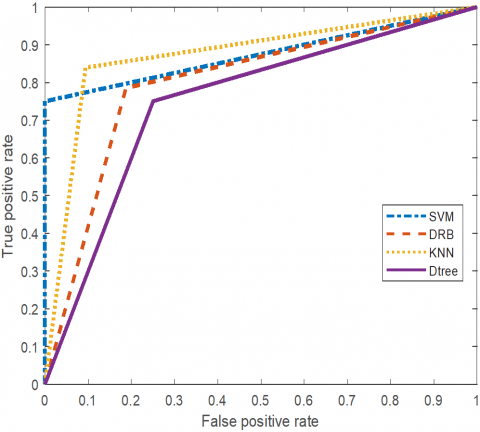Dataset 1

## 7-2.png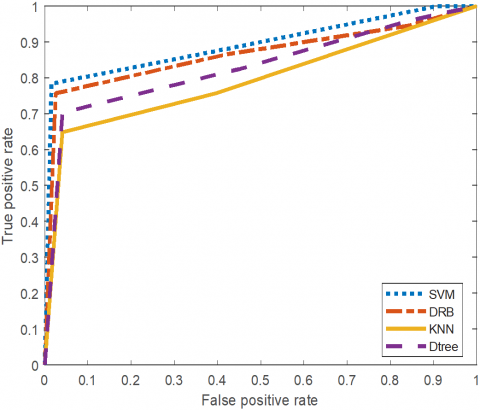Dataset 2

Figure 7. ROC curves of dataset 1 and dataset 2

Experiment 3: ResNet-50 with 4 different classifiers.

## 8-1.pngDataset 1

## 8-2.pngDataset 2

Figure 8. Confusion matrix of DRB with data 1 and data 2

## 9-1.pngDataset 1

## 9-2.png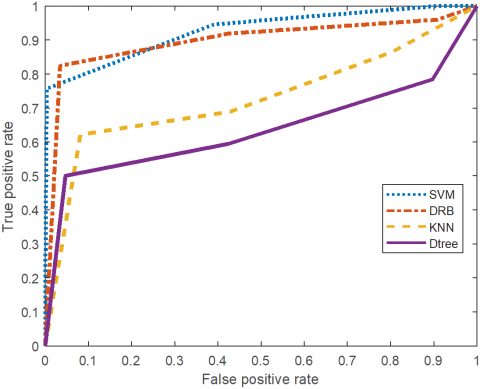Dataset 2

Figure 9. ROC curves of dataset 1 and dataset 2

Experiment 4: ResNet-18 with 4 different classifiers.

## 10-.png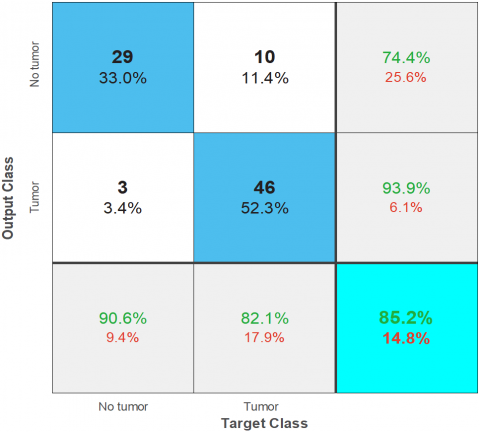Dataset 1

## 10-2.png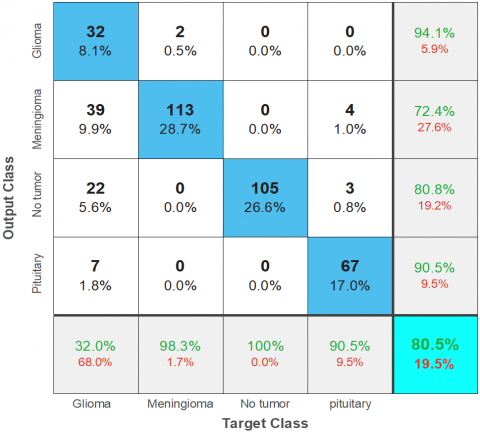Dataset 2

Figure 10. Confusion matrix of DRB with data 1 and data 2

## 11-1.pngDataset 1

## 11-2.png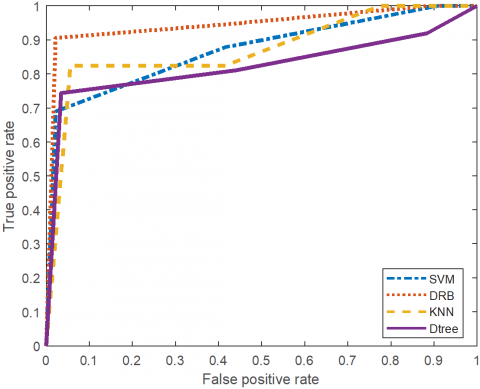Dataset 2

Figure 11. ROC curves of dataset 1 and dataset 2

Table 5. Comparative performance of AlexNet with 4 different classifiers

 AlexNet with 4 different classifiers Data set Architecture Accuracy Sensitivity Specificity Precision F-measure Dataset1 DRB SVM KNN Decision Tree 85.23% 79.55% 89.77% 69.32% 0.9375 0.8438 0.8750 0.6563 0.8036 0.7679 0.9107 0.7143 0.7317 0.6750 0.8485 0.5676 0.8219 0.7500 0.8615 0.6087 Dataset2 DRB SVM KNN Decision Tree 79.19% 75.63% 60.66% 71.32% 0.3500 0.2000 0.3700 0.4000 0.9422 0.9456 0.6871 0.8197 0.6131 0.5556 0.2868 0.4301 0.4605 0.2941 0.3231 0.4145

Table 6. Comparative performance of VGG-16 with 4 different classifiers

 VGG-16 with 4 different classifiers Dataset Architecture Accuracy Sensitivity Specificity Precision F-measure Dataset1 DRB SVM KNN Decision Tree 79.55% 84.09% 86.36% 75.00% 0.8125 0.7500 0.9063 0.7500 0.7857 1 0.8393 0.7500 0.6842 0.6957 0.7632 0.6316 0.7429 0.8205 0.8286 0.6857 Dataset2 DRB SVM KNN Decision Tree 81.73% 77.16% 61.93% 72.84% 0.4800 0.2900 0.4400 0.3800 0.9422 0.9456 0.6871 0.8197 0.7059 0.6042 0.3188 0.4578 0.5714 0.3919 0.3697 0.4153

Table 7. Comparative performance of ResNet 50 with 4 different classifiers

 ResNet-50 with 4 different classifiers Dataset Architecture Accuracy Sensitivity Specificity Precision F-measure Dataset1 DRB SVM KNN Decision Tree 82.95% 78.41% 79.55% 72.73% 0.8125 0.8125 0.8750 0.7188 0.8393 0.7679 0.7500 0.7321 0.7429 0.6667 0.6667 0.6053 0.7761 0.7324 0.7568 0.6571 Dataset2 DRB SVM KNN Decision Tree 78.17% 77.16 % 62.18 % 68.78 % 0.2900 0.3000 0.4100 0.2800 0.9490 0.9320 0.6939 0.8265 0.6591 0.6000 0.3130 0.3544 0.4028 0.4000 0.3550 0.3128

Table 8. Comparative performance of ResNet18 with 4 different classifiers

 ResNet18 with 4 different classifiers Dataset Architecture Accuracy Sensitivity Specificity Precision F-measure Dataset1 DRB SVM KNN Decision Tree 85.22% 77.27% 81.82% 69.32% 0.9063 0.6250 0.7813 0.9063 0.8214 0.8571 0.8393 0.7514 0.7436 0.7143 0.7353 0.5472 0.8169 0.6667 0.7576 0.6824 Dataset2 DRB SVM KNN Decision Tree 80.46% 75.89% 65.74% 73.35% 0.3200 0.2900 0.3900 0.3200 0.9694 0.9184 0.7483 0.8741 0.7805 0.5472 0.3451 0.4638 0.4539 0.3791 0.3662 0.3787
6. Conclusion

In this study, we have presented an automated MRI brain tumor identification technique using deep learning methods. The framework employed the DRB classifier to perform the MRI brain tumor classification tasks. The deep features were extracted by deep learning models such as AlexNet, ResNet-50, ResNet-18, and VGG-16. The developed method was evaluated on two datasets provided on Kaggle website. The first dataset is a binary database (i.e., tumor, no tumor), while the second one is a multiclass database (i.e., Meningioma, Glioma, and Pituitary tumor). The experimental results showed that the devised method based on DRB classifier for tumor classification is a robust, simple, and competent, which can reach high level of performance. An accuracy of 79.19% was obtained with AlexNet, 81.73% with VGG-16, 78.17% with ResNet50, and 80.46% with ResNet18 surpassing SVM, KNN and decision tree techniques. It is hoped that this framework will help neuroscientist in their job, which is most difficult task, i.e., making decisions from MRI brain data, and to avoid wrong judgments on subjects.

In the future, we will explore two main directions. First, we will extend the devised framework with other specific modules for detection of other and numerous pathological diseases, second, open-source web-based systems that could be freely used by medical practitioners and researchers.

References

 Lodge, M. (2020). The role of the Commonwealth in the wider cancer control agenda. The Lancet Oncology, 21(7): 879-881. https://doi.org/10.1016/S1470-2045(20)30222-9

 Badža, M.M., Barjaktarović, M.Č. (2020). Classification of brain tumors from MRI images using a convolutional neural network. Applied Sciences, 10(6): 1999. https://doi.org/10.3390/app10061999

 Burçak, K.C., Uğuz, H. (2022). A new hybrid breast cancer diagnosis model using deep learning model and ReliefF. Traitement du Signal, 39(2): 521-529. https://doi.org/10.18280/ts.390214

 Bulla, P., Anantha, L., Peram, S. (2020). Deep neural networks with transfer learning model for brain tumors classification. Traitement du Signal, 37(4): 593-601. https://doi.org/10.18280/ts.370407

 Attia, A., Moussaoui, A., Chahir, Y. (2017). An EEG-fMRI fusion analysis based on symmetric techniques using dempster shafer theory. Journal of Medical Imaging and Health Informatics, 7(7): 1493-1501. https://doi.org/10.1166/jmihi.2017.2185

 Varuna S.N., Kumar, T.N.R. (2018). Identification and classification of brain tumor MRI images with feature extraction using DWT and probabilistic neural network. Brain Informatics, 5(1): 23-30. https://doi.org/10.1007/s40708-017-0075-5

 Cheng, J., Huang, W., Cao, S., Yang, R., Yang, W., Yun, Z., Wang, Z., Feng, Q. (2015). Enhanced performance of brain tumor classification via tumor region augmentation and partition. PloS One, 10(10): e0140381. https://doi.org/10.1371/journal.pone.0140381

 Zhang, Y.D., Wang, S.H., Yang, X.J., Dong, Z.C., Liu, G., Phillips, P., Yuan, T.F. (2015). Pathological brain detection in MRI scanning by wavelet packet Tsallis entropy and fuzzy support vector machine. SpringerPlus, 4(1): 1-16. https://doi.org/10.1186/s40064-015-1523-4

 Bahadure, N.B., Ray, A.K., Thethi, H.P. (2017). Image analysis for MRI based brain tumor detection and feature extraction using biologically inspired BWT and SVM. International Journal of Biomedical Imaging, 2017: 9749108. https://doi.org/10.1155/2017/9749108

 Zhang, Y.D., Wu, L. (2012). An MR brain images classifier via principal component analysis and kernel support vector machine. Progress In Electromagnetics Research, 130: 369-388. http://dx.doi.org/10.2528/PIER12061410

 Usman, K., Rajpoot, K. (2017). Brain tumor classification from multi-modality MRI using wavelets and machine learning. Pattern Analysis and Applications, 20: 871-881. https://doi.org/10.1007/s10044-017-0597-8

 Ari, A., Hanbay, D. (2018). Deep learning based brain tumor classification and detection system. Turkish Journal of Electrical Engineering and Computer Sciences, 26(5): 2275-2286. https://doi.org/10.3906/elk-1801-8

 Byale, H., Lingaraju, G.M., Sivasubramanian, S. (2018). Automatic segmentation and classification of brain tumor using machine learning techniques. International Journal of Applied Engineering Research, 13(14): 11686-11692.

 Krizhevsky, A., Sutskever, I., Hinton, G.E. (2017). Imagenet classification with deep convolutional neural networks. Communications of the ACM, 60(6): 84-90. https://doi.org/10.1145/3065386

 Bengio, Y. (2009). Learning deep architectures for AI. Foundations and trends® in Machine Learning, 2(1): 1-127. http://dx.doi.org/10.1561/2200000006

 Szegedy, C., Liu, W., Jia, Y., Sermanet, P., Reed, S., Anguelov, D., Erhan, D., Vanhoucke, V., Rabinovich, A. (2015). Going deeper with convolutions. In Proceedings of the IEEE Conference on Computer Vision and Pattern Recognition pp. 1-9.

 Simonyan, K., Zisserman, A. (2014). Very deep convolutional networks for large-scale image recognition. arXiv preprint arXiv:1409.1556. https://doi.org/10.48550/arXiv.1409.1556

 He, K., Zhang, X., Ren, S., Sun, J. (2016). Deep residual learning for image recognition. In Proceedings of the IEEE Conference on Computer Vision and Pattern Recognition, pp. 770-778.

 Xie, S., Girshick, R., Dollár, P., Tu, Z., He, K. (2017). Aggregated residual transformations for deep neural networks. In Proceedings of the IEEE Conference on Computer Vision and Pattern Recognition, pp. 1492-1500.

 Angelov, P.P., Gu, X. (2018). Deep rule-based classifier with human-level performance and characteristics. Information Sciences, 463: 196-213. https://doi.org/10.1016/j.ins.2018.06.048

 Angelov, P. (2012). Autonomous Learning Systems: From Data Streams to Knowledge in Real-Time. John Wiley & Sons.

 Angelov, P.P., Zhou, X. (2008). Evolving fuzzy-rule-based classifiers from data streams. IEEE Transactions on Fuzzy Systems, 16(6): 1462-1475. https://doi.org/10.1109/TFUZZ.2008.925904

 Angelov, P., Yager, R. (2012). A new type of simplified fuzzy rule-based system. International Journal of General Systems, 41(2): 163-185. https://doi.org/10.1080/03081079.2011.634807

 Angelov, P.P., Gu, X., Príncipe, J.C. (2017). A generalized methodology for data analysis. IEEE Transactions on Cybernetics, 48(10): 2981-2993. https://doi.org/10.1109/TCYB.2017.2753880

 Angelov, P., Gu, X. (2017). Autonomous learning multi-model classifier of 0-order (ALMMo-0). In 2017 Evolving and Adaptive Intelligent Systems (EAIS), Ljubljana, Slovenia, pp. 1-7. https://doi.org/10.1109/EAIS.2017.7954832

 Handelman, G.S., Kok, H.K., Chandra, R.V., Razavi, A.H., Huang, S., Brooks, M., Lee, M.J., Asadi, H. (2019). Peering into the black box of artificial intelligence: evaluation metrics of machine learning methods. American Journal of Roentgenology, 212(1): 38-43. https://doi.org/10.2214/AJR.18.20224